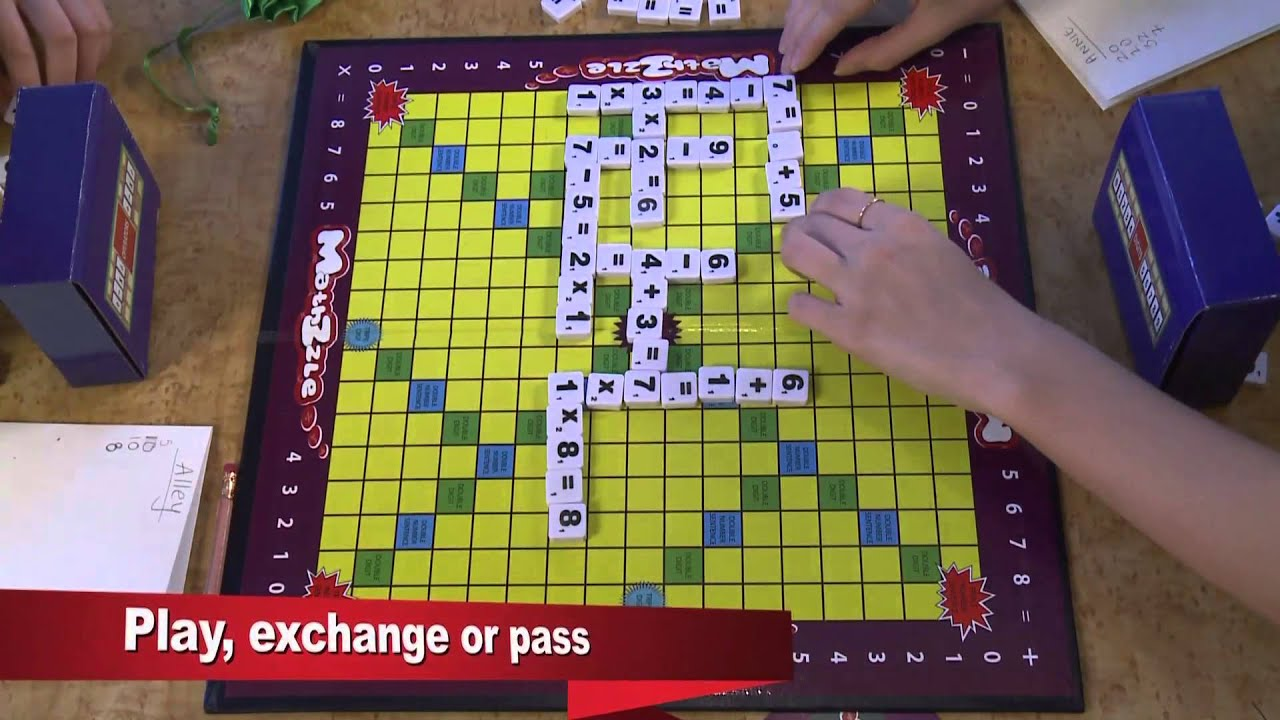# Mathzzle! How-to play math board game

0
7Mathzzle: Where Math Meets Fun! New math board game for the whole family. Awarded Gold Medal in the Belgian & Intl. Trade Fair for Technological Innovation.
MATHZZLE: Where Math meets fun!

In Mathzzle, players earn points by forming complete number sentences or equations with tiles and placing them on a grid.

Step 1: Draw 8 tiles
Players randomly pick tiles from the 3 compartments, choosing 5
Whole numbers (0-9), 2 Operators (+, −, x, and ÷) and 1 Equal sign ( =).
For a total of 8 tiles.

Step 2:
The first player begins the game by placing an equation across the “M” square at the center of the board, either horizontally or vertically.
This “M” acts as a double-equation score.

Play
The direction of the equation can be either across (left to right) or down (top to down) . Reverse directions are not allowed.

Step 3: Score each equation
Tally each turn by adding point values for each square in the equation.

Landing on colored squares allow you to multiply the tile or the entire number sentence value by two or three.

Any digit that is placed on the “Double Number Sentence” square will get double the total score for an equation. This score is tripled when one digit of its equation is placed on the “Triple Number Sentence” square. If an equation is formed that covers two colored digit squares, the score is doubled and re-doubled, or tripled and then re-tripled.

Tip
Some colored squares on the board allow you to multiply the tile or equation’s value by two or three.

For example:
(ii) 5 + 5 = 10 (the value of digit “5” is one, “+” symbol is one, “=” is one, “1” and “0” also one, the total is 1+1+1+1+1+1 = 6)
(iii) 5 + 5 = 10 (If the equation formed hits the colored square “Double Number Sentence”, the total will be 6 [refer to e.g. 4.(i)] x 2 = 12)
(iv) If the same equation hits the colored square “Double Number Sentence” twice, the total will be 12 [refer to e.g. 4.(ii)] x 2 = 24)

Step 4: Continue play
When placing equations on the board, all tiles must touch at least one other tile already on the board to form at least one complete number sentence.

During each turn, a player can decide to place an equation on the board, exchange tiles, or pass.

If a player decides to exchange tiles they cannot do anything else on that turn.

Step 5: Choose new tiles
After placing an equation on the board, draw new tiles so that each player always has 8 tiles.

Step 6: JACKPOT!
If a player plays ALL 8 tiles on a turn, it’s a Jackpot! The player adds a 40- point bonus to their equation for that turn.

Step 7: Continue until game ends
When one player has used all of their tiles or no one can make any more equations, the game ends.

Step 8: Calculate the scores
Calculate each player’s final score by adding up the points earned during play. Then, subtract the sum of their un-played tiles.
The player with the highest score wins.

Nguồn: https://trulyluminary.com/

Xem thêm bài viết khác: https://trulyluminary.com/game/